Question

2. A beam with a uniform flexural rigidity, EI, is loaded by a triangular distributed load,...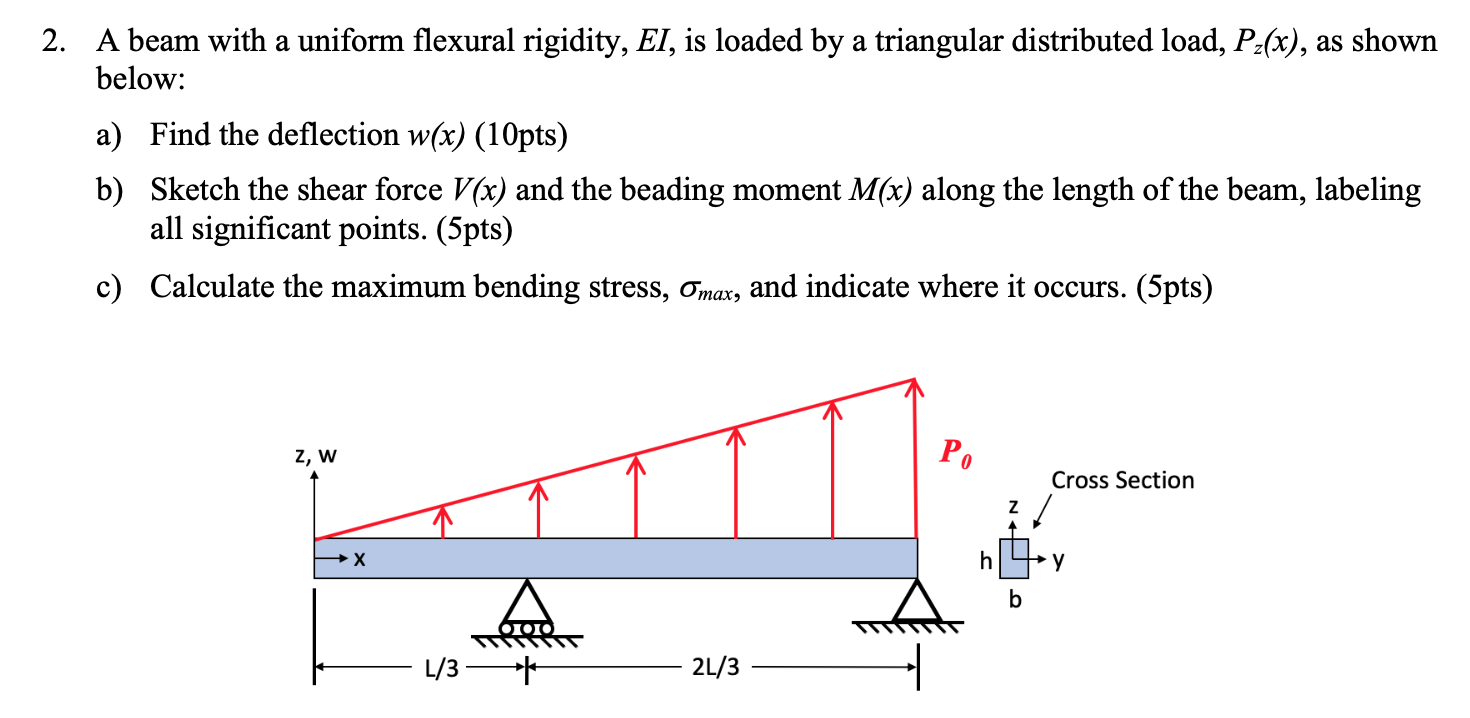2. A beam with a uniform flexural rigidity, EI, is loaded by a triangular distributed load, Pz(x), as shown below: a) Find the deflection w(x) (10pts) b) Sketch the shear force V(x) and the beading moment M(x) along the length of the beam, labeling all significant points. (5pts) c) Calculate the maximum bending stress, Omax, and indicate where it occurs. (5pts) z, W Cross Section - 1/3 — * - 2/3 —

Given that :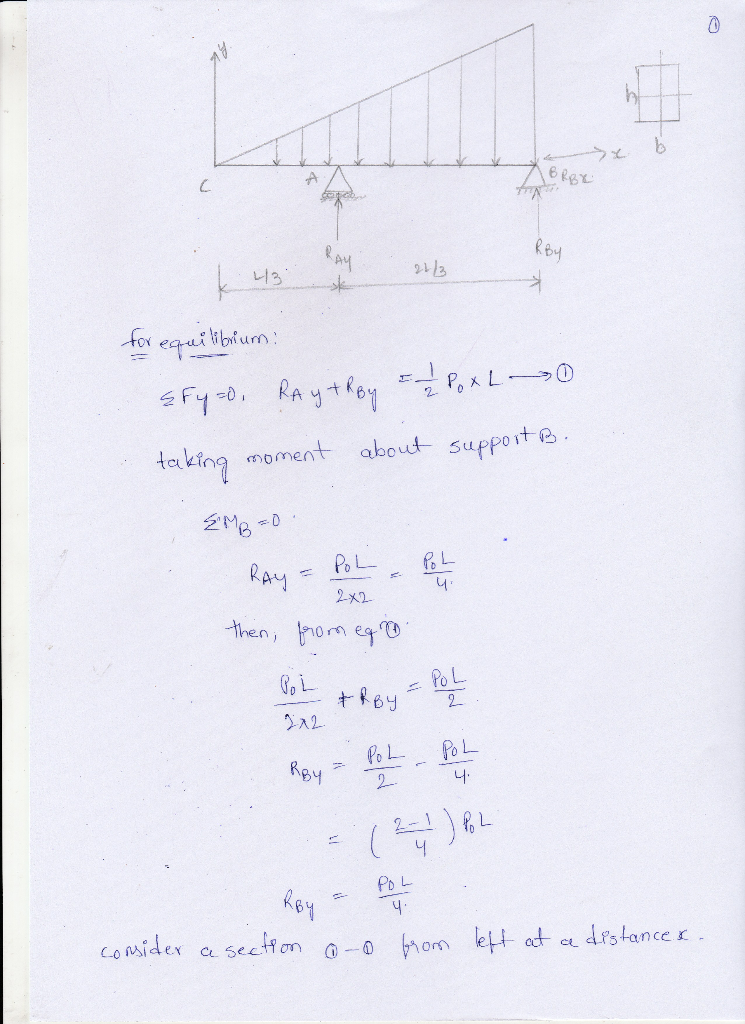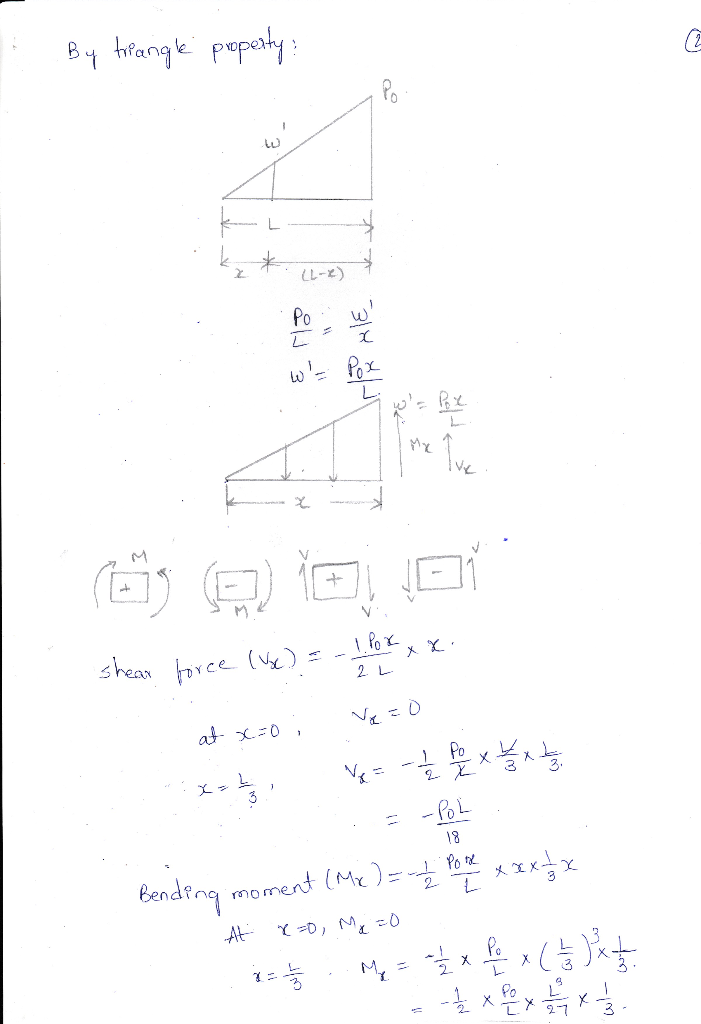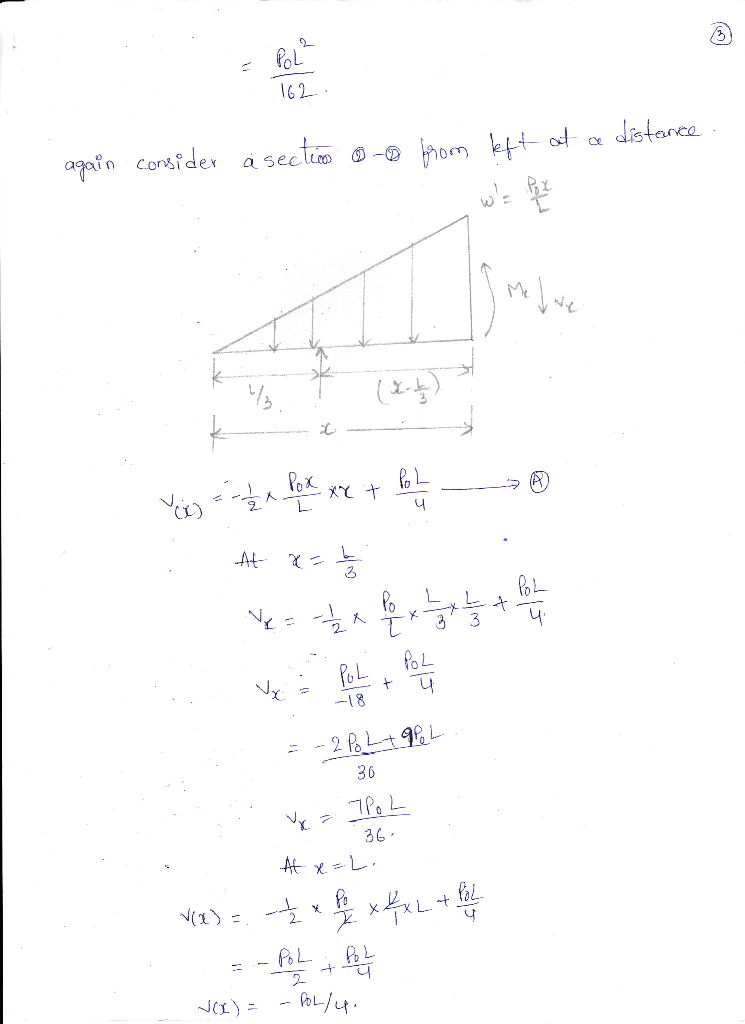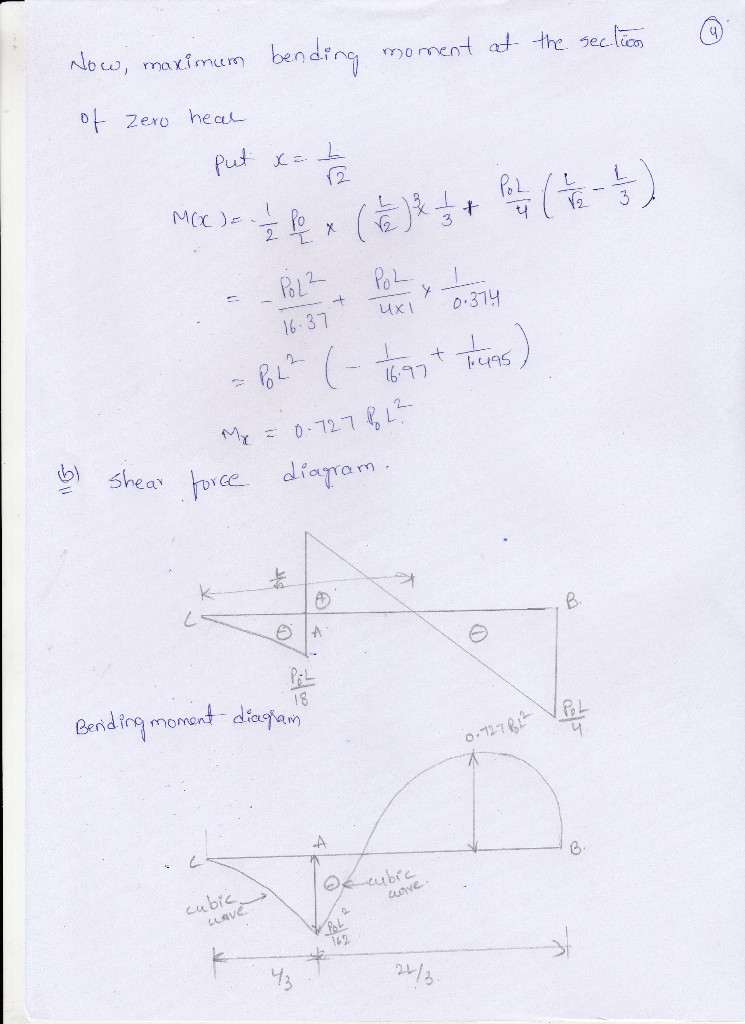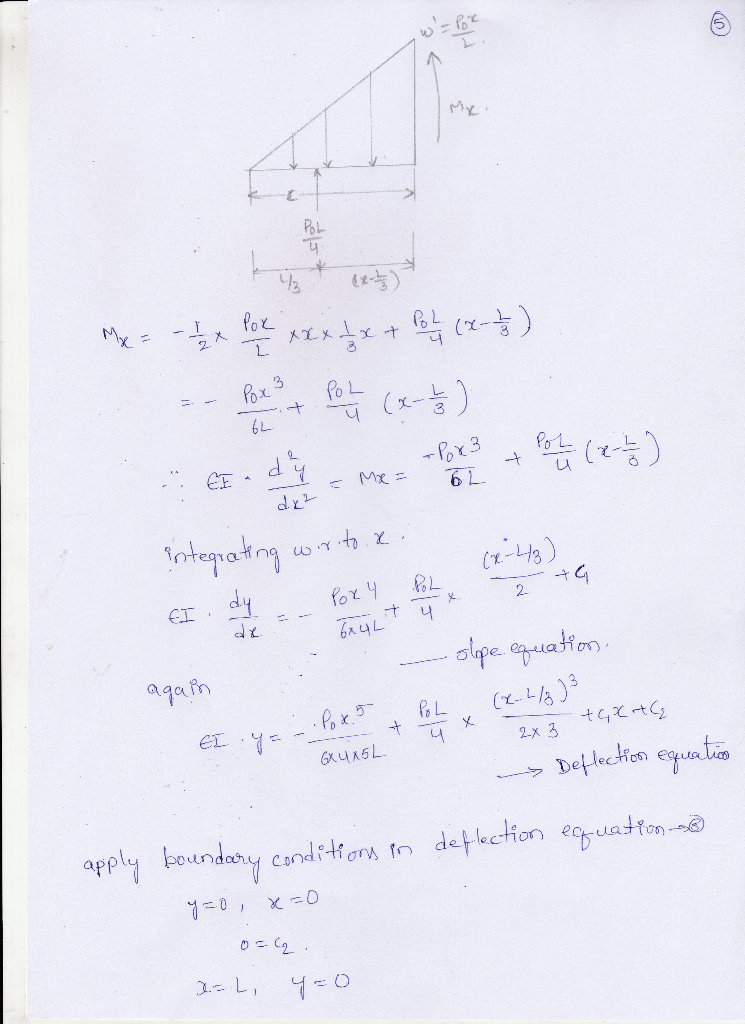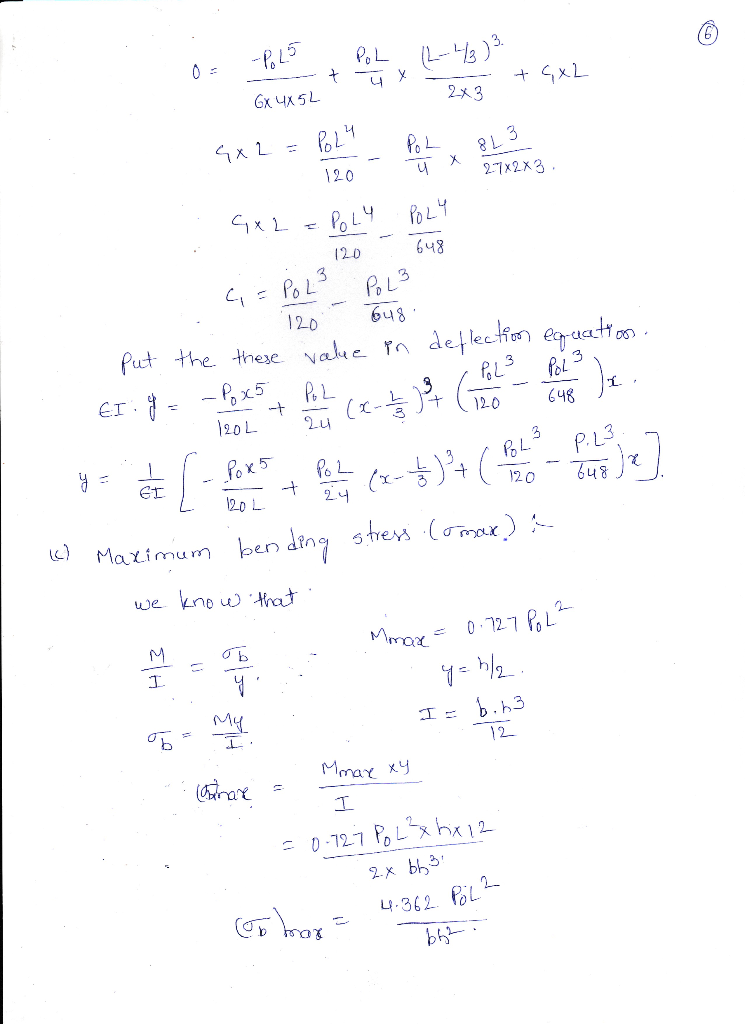Earn Coins

Coins can be redeemed for fabulous gifts.

Similar Homework Help Questions
• Choose the correct statement. The shear load is the product of the flexural rigidity (EI) and...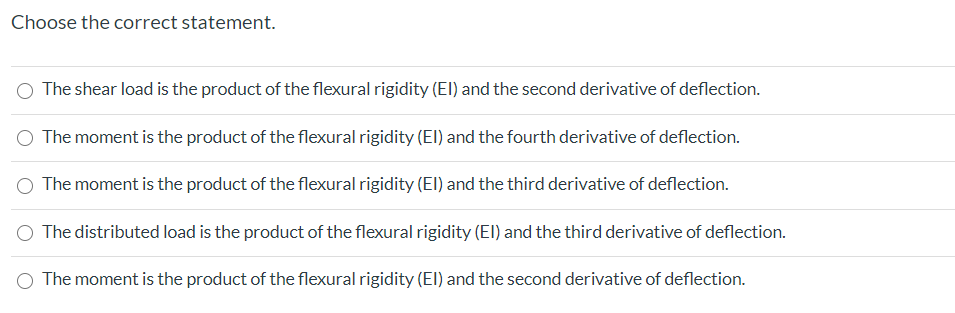Choose the correct statement. The shear load is the product of the flexural rigidity (EI) and the second derivative of deflection. The moment is the product of the flexural rigidity (El) and the fourth derivative of deflection. The moment is the product of the flexural rigidity (EI) and the third derivative of deflection. The distributed load is the product of the flexural rigidity (El) and the third derivative of deflection. The moment is the product of the flexural rigidity (El)...

• The cantilevered beam shown here has a known rigidity, EI, and length, b, and is loaded...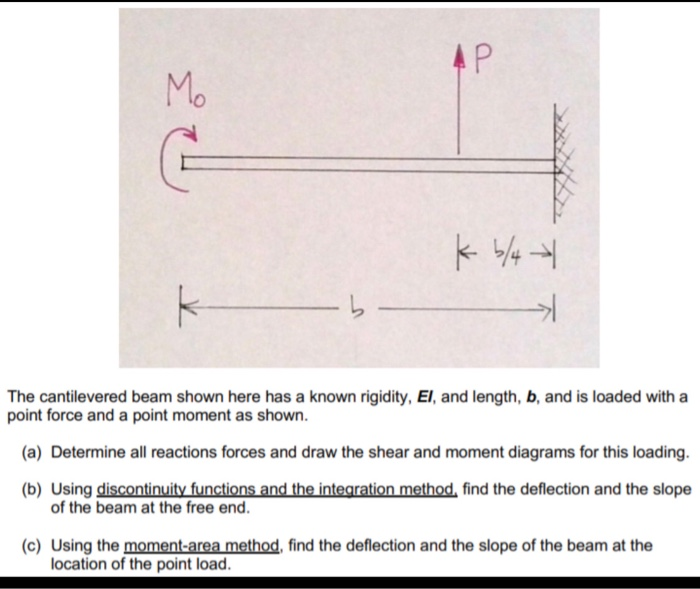The cantilevered beam shown here has a known rigidity, EI, and length, b, and is loaded with a point force and a point moment as shown a) Determine all reactions forces and draw the shear and moment diagrams for this loading. b) Using discontinuity functions and the integration method, find the deflection and the slope of the beam at the free end. c) Using the moment-area method, find the deflection and the slope of the beam at the location of...

• The cantilevered beam shown here has a known rigidity, EI, and length, b, and is loaded...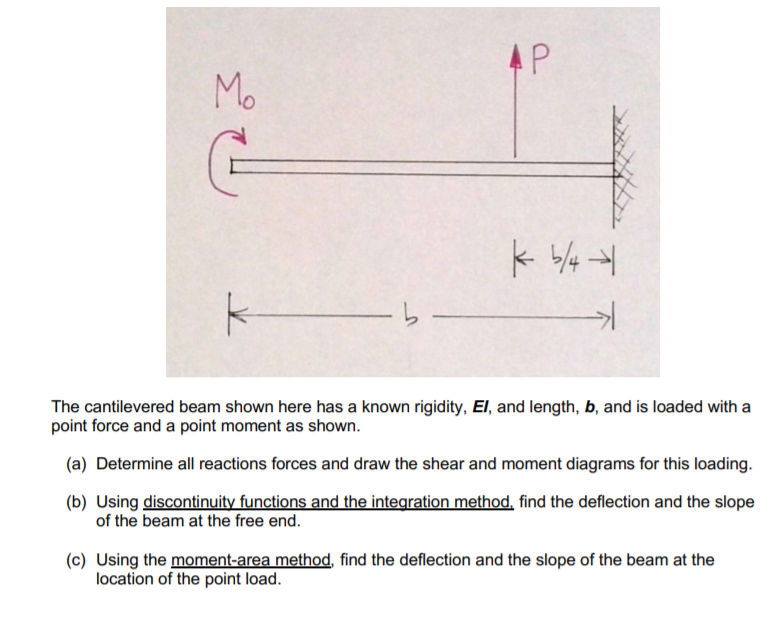The cantilevered beam shown here has a known rigidity, EI, and length, b, and is loaded with a point force and a point moment as shown. (a) Determine all reactions forces and draw the shear and moment diagrams for this loading. (b) Using discontinuity functions and the integration method, find the deflection and the slope of the beam at the free end. (c) Using the moment-area method, find the deflection and the slope of the beam at the location of...

• QUESTION 4 (25 marks) A simply supported beam is loaded by an uniform distributed load, wkN/m, over the span of the beam, L, as shown in Figure Q4. (a) Determine the end reactions at point A and...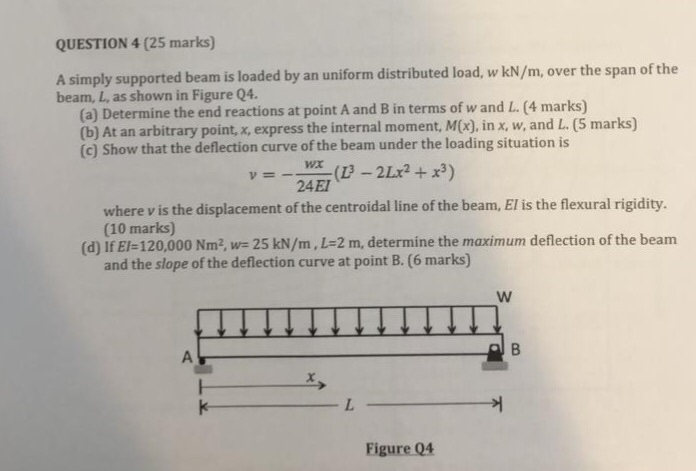QUESTION 4 (25 marks) A simply supported beam is loaded by an uniform distributed load, wkN/m, over the span of the beam, L, as shown in Figure Q4. (a) Determine the end reactions at point A and B in terms of w and L. (4 marks) (b) At an arbitrary point, x, express the internal mom (c) Show that the deflection curve of the beam under the loading situation is ent, M(x), in x, w, and L. (5 marks) 24EI...

• 4. (25 pt.) The beam subjected to a uniform distributed load as shown in Figure 4(a)...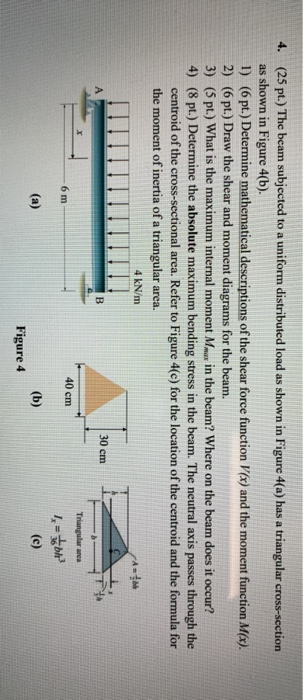4. (25 pt.) The beam subjected to a uniform distributed load as shown in Figure 4(a) has a triangular cross-section as shown in Figure 4(b). 1) (6 pt.) Determine mathematical descriptions of the shear force function V(x) and the moment function M(x). 2) (6 pt.) Draw the shear and moment diagrams for the beam. 3) (5 pt.) What is the maximum internal moment Mmar in the beam? Where on the beam does it occur? 4) (8 pt.) Determine the absolute...

• The below wooden double overhanging beam is under a uniformly distributed load W. The wood is...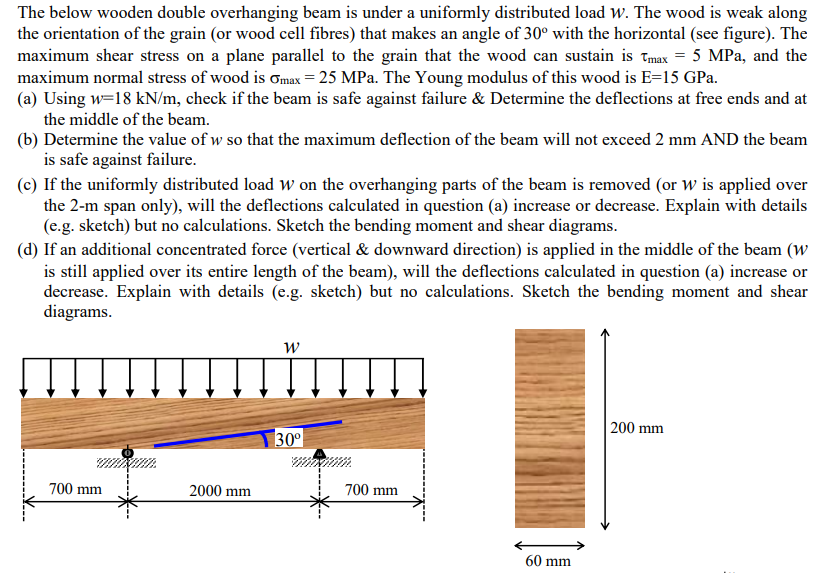The below wooden double overhanging beam is under a uniformly distributed load W. The wood is weak along the orientation of the grain (or wood cell fibres) that makes an angle of 30° with the horizontal (see figure). The maximum shear stress on a plane parallel to the grain that the wood can sustain is t,max = 5 MPa, and the maximum normal stress of wood is omax = 25 MPa. The Young modulus of this wood is E=15 GPa....

• (2) A simply supported beam of flexural rigidity El carries a constant uniformly distributed load of...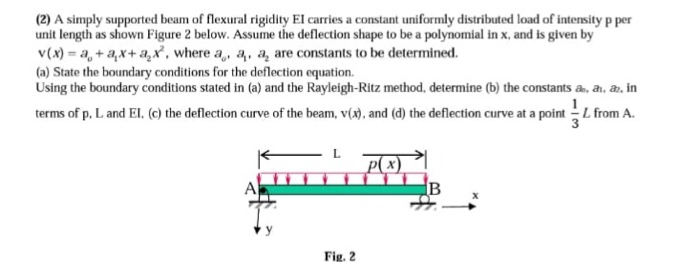(2) A simply supported beam of flexural rigidity El carries a constant uniformly distributed load of intensity p per unit length as shown Figure 2 below. Assume the deflection shape to be a polynomial in x, and is given by v (x) = a., + as+ a2 x, where ao, a.呙are constants to be determined. (a) State the boundary conditions for the deflection equation. Using the boundary conditions stated in (a) and the Rayleigh-Ritz method, determine (b) the constants a,...

• The W33 x221 steel simply supported beam is loaded with concentrated loads and uniform load as...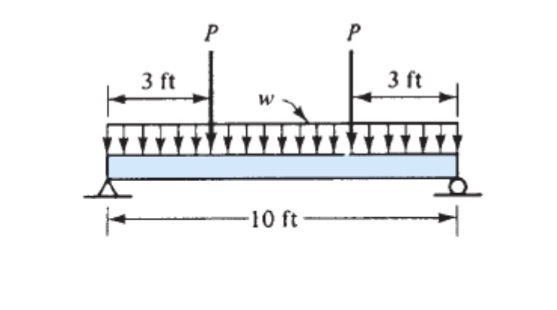The W33 x221 steel simply supported beam is loaded with concentrated loads and uniform load as shown with the load P= 150kip and w = 10kip/ft. For this beam do the following; a) Draw the shear and bending moment diagram b) Calculate the maximum compressive and tensile stress c) Calculate the maximum shear stress P Р 3 ft 3 ft w 10 ft

• 4N Problem 6. The beam shown is loaded with a linear distributed load for the left...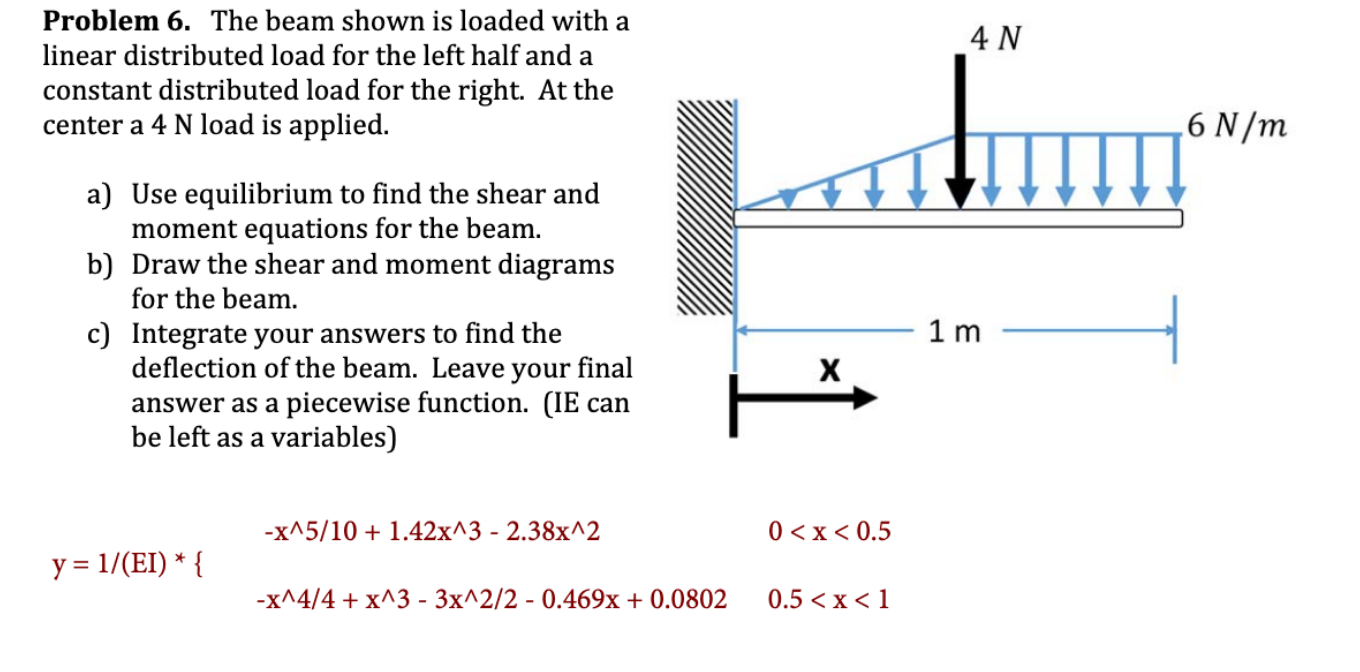4N Problem 6. The beam shown is loaded with a linear distributed load for the left half and a constant distributed load for the right. At the center a 4 N load is applied. 6 N/m a) Use equilibrium to find the shear and moment equations for the beam. b) Draw the shear and moment diagrams for the beam. c) Integrate your answers to find the deflection of the beam. Leave your final answer as a piecewise function. (IE can...

• Question 1 1. A steel beam is loaded as shown complete the following a. Draw a...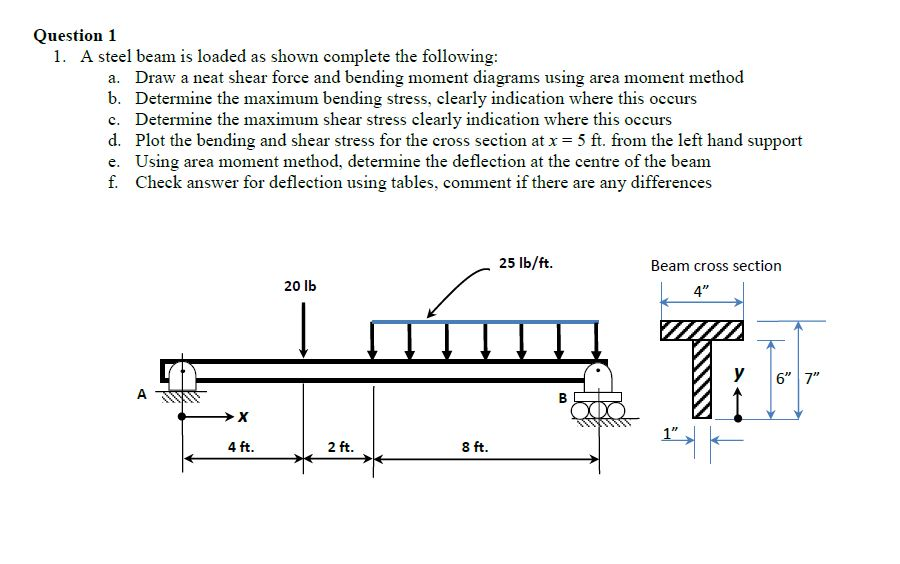Question 1 1. A steel beam is loaded as shown complete the following a. Draw a neat shear force and bending moment diagrams using area moment method b. Determine the maximum bending stress, clearly indication where this occurs c. Determine the maximum shear stress clearly indication where this occurs d, Plot the bending and shear stress for the cross section at x = 5 ft. from the left hand support e. Using area moment method, determine the deflection at the...